Question

# Two people with masses 55 kg and 72 kg stand 3.2 m apart. What is the...

1. Two people with masses 55 kg and 72 kg stand 3.2 m apart. What is the magnitude of the

gravitational attraction between them? G = 6.674 x 10^-11

Let's

Masses -

m1​​​​​​ = 55 kg

m2​​​​​​ = 72 kg

Distance (r) = 3.2 meter

Gravitational force between two masses -

F = Gm1m2/r2

F = (6.674 x 10-11x 55 x72) /(3.22​​​​​)

F = 2.581 x​​​​​​ 10-8​​​​​​8 Newton

#### Earn Coins

Coins can be redeemed for fabulous gifts.

Similar Homework Help Questions
• ### 12. Two rocks, each of mass 72 kg, are positioned 95 m away from each other...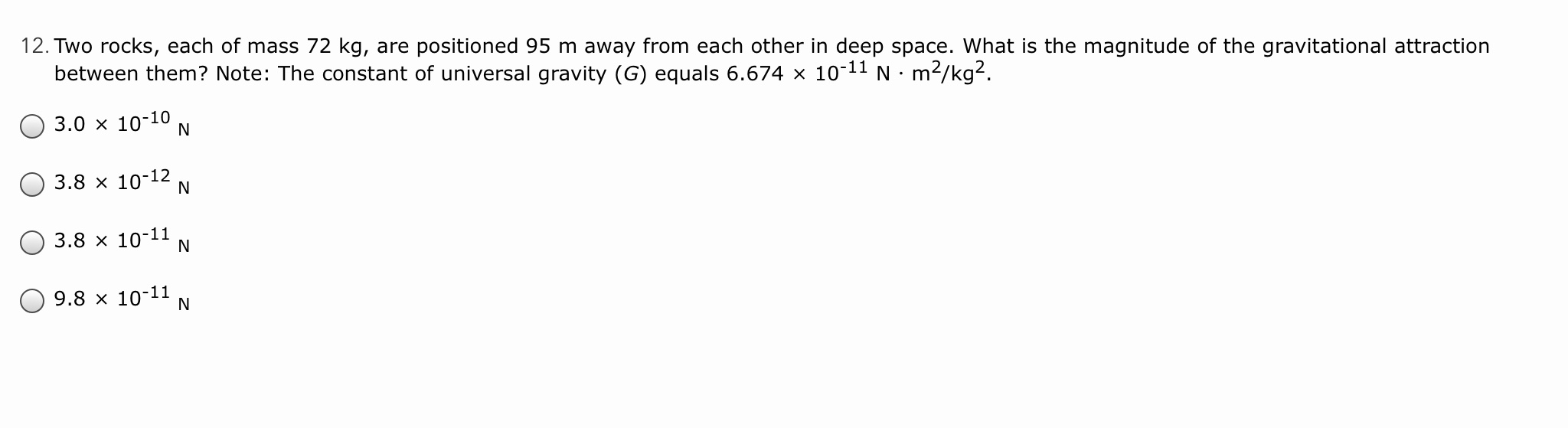12. Two rocks, each of mass 72 kg, are positioned 95 m away from each other in deep space. What is the magnitude of the gravitational attraction between them? Note: The constant of universal gravity (G) equals 6.674 10-11 N·m2/kg2. O 3.0 10-10 N O3.8 x 10-12 N O3.8 x 10-11 N O9.8 x 10-11 N

• ### 3) Two 1.0 kg masses are 1.0 m apart (center to center) on a frictionless table....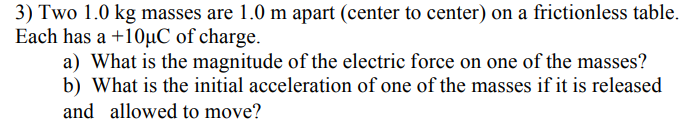3) Two 1.0 kg masses are 1.0 m apart (center to center) on a frictionless table. Each has a +10uC of charge. a) What is the magnitude of the electric force on one of the masses? b) What is the initial acceleration of one of the masses if it is release<d and allowed to move?

• ### A heavier mass m1 and a lighter mass m2 are 19.5 cm apart and experience a...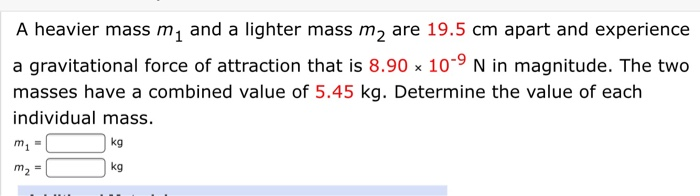A heavier mass m1 and a lighter mass m2 are 19.5 cm apart and experience a gravitational force of attraction that is 8.90 x 10-9 N in magnitude. The two masses have a combined value of 5.45 kg. Determine the value of each individual mass. m₂ = kg m₂ = kg

• ### For two objects with masses 100kg and 500 kg to be 20 meters apart, find the...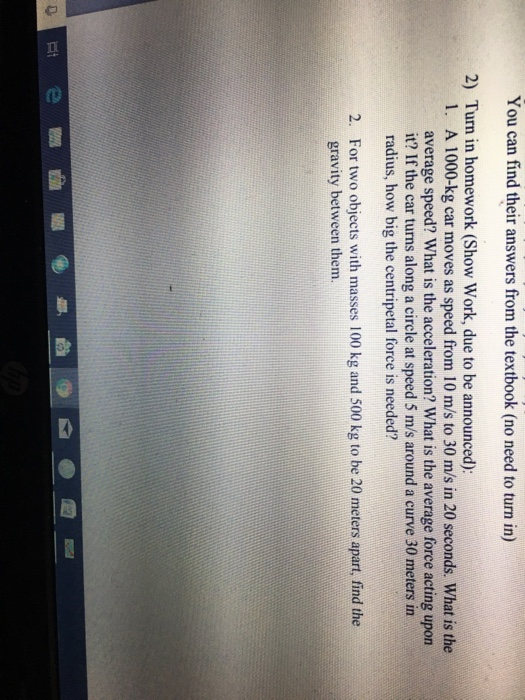For two objects with masses 100kg and 500 kg to be 20 meters apart, find the gravity between them. You can find their answers from the textbook (no need to turn in) 2) Turn in homework (Show Work, due to be announced): A 1000-kg car moves as speed from 10 m/s to 30 m/s in 20 seconds. What is the average speed? What is the acceleration? What is the average force acting upon it? If the car turns along a...

• ### Objects with masses of 222 kg and 212 kg are separated by 0.375 m. A 69.8...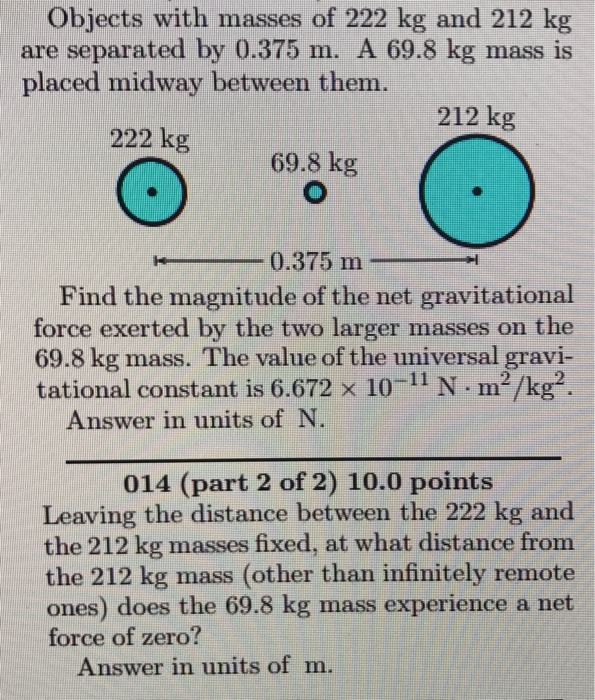Objects with masses of 222 kg and 212 kg are separated by 0.375 m. A 69.8 kg mass is placed midway between them 212 kg 222 kg 69.8 kg 0.375 m Find the magnitude of the net gravitational force exerted by the two larger masses on the 69.8 kg mass. The value of the universal gravi- tational constant is 6.672 × 10-11 N·m2/kg2 Answer in units of N 014 (part 2 of 2) 10.0 points Leaving the distance between the...

• ### Two 639-kg masses are separated by a distance of 0.15 m.  Using Newton's Law of Universal Gravitation,...

Two 639-kg masses are separated by a distance of 0.15 m.  Using Newton's Law of Universal Gravitation, find the gravitational force of attraction between these two masses.

• ### Two 3.7 kg physical science textbooks on a bookshelf are 0.33 mapart. What is the...

Two 3.7 kg physical science textbooks on a bookshelf are 0.33 m apart. What is the magnitude of the gravitational attraction between the books?

• ### Part A: Objects with masses of 81 kg and 634 kg are separated by 0.362 m....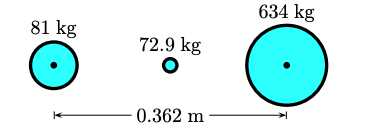Part A: Objects with masses of 81 kg and 634 kg are separated by 0.362 m. A 72.9 kg mass is placed midway between them.Find the magnitude of the net gravitational force exerted by the two larger masses on the 72.9 kg mass. The value of the universal gravitational constant is 6.672 × 10−11 N · m2 /kg2 . Answer in units of N. Part B: Leaving the distance between the 81 kg and the 634 kg masses fixed, at...

• ### Estimate the gravitational force (in N) between two sumo wrestlers, with masses 235 kg and 237...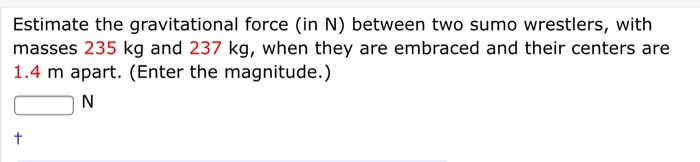Estimate the gravitational force (in N) between two sumo wrestlers, with masses 235 kg and 237 kg, when they are embraced and their centers are 1.4 m apart. (Enter the magnitude.) ON

• ### Two spherical objects have a combined mass of 200 kg. The gravitational attraction between them is...

Two spherical objects have a combined mass of 200 kg. The gravitational attraction between them is 1.5174 x 10-5N when their centers are 20 cm apart. What is the mass of each object? a. 100 and 100 kg b. 130 and 70 kg c. 145 and 55 kg d. 160 and 40 kg e. 185 and 15 kg